Next: Simple Harmonic Oscillator Equation Up: Simple Harmonic Oscillation Previous: Simple Harmonic Oscillation

# Mass on a Spring

Consider a compact mass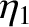that slides over a frictionless horizontal surface. Suppose that the mass is attached to one end of a light horizontal spring whose other end is anchored in an immovable wall. (See Figure 1.) At time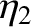, let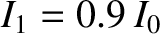be the extension of the spring: that is, the difference between the spring's actual length and its unstretched length.can also be used as a coordinate to determine the instantaneous horizontal displacement of the mass.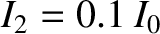The equilibrium state of the system corresponds to the situation in which the mass is at rest, and the spring is unextended (i.e.,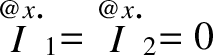, where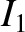). In this state, zero horizontal force acts on the mass, and so there is no reason for it to start to move. However, if the system is perturbed from its equilibrium state (i.e., if the mass is displaced sideways, such that the spring becomes extended) then the mass experiences a horizontal force given by Hooke's law,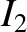(1)

Here,is the so-called force constant of the spring. The negative sign in the preceding expression indicates thatis a restoring force (i.e., if the displacement is positive then the force is negative, and vice versa). The magnitude of this restoring force is directly proportional to the displacement of the mass from its equilibrium position (i.e.,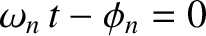). Hooke's law only holds for relatively small spring extensions. Hence, the displacement of the mass from its equilibrium position cannot be made too large, otherwise Equation (1) ceases to be valid. Incidentally, the motion of this particular dynamical system is representative of the motion of a wide variety of different mechanical systems when they are slightly disturbed from a stable equilibrium state. (See Section 2.4.)

Newton's second law of motion leads to the following time evolution equation for the system,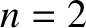(2)

where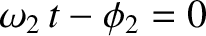. This differential equation is known as the simple harmonic oscillator equation, and its solution has been known for centuries. The solution is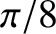(3)

where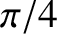,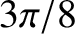, andare constants. We can demonstrate that Equation (3) is indeed a solution of Equation (2) by direct substitution. Plugging the right-hand side of Equation (3) into Equation (2), and recalling from standard calculus that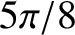and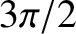(see Appendix B), so that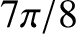and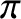, where use has been made of the chain rule,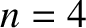(4)

we obtain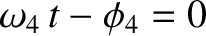(5)

It follows that Equation (3) is the correct solution provided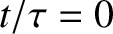(6)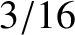Figure 2 shows a graph of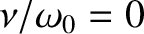versusderived from Equation (3). The type of motion displayed here is called simple harmonic oscillation. It can be seen that the displacementoscillates between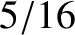and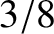. Here,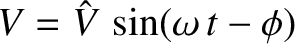is termed the amplitude of the oscillation. Moreover, the motion is repetitive in time (i.e., it repeats exactly after a certain time period has elapsed). The repetition period is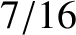(7)

This result can be obtained from Equation (3) by noting that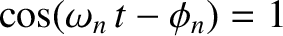is a periodic function of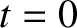with period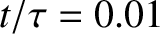: that is,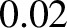. It follows that the motion repeats each time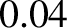increases by. In other words, each timeincreases by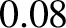. The frequency of the motion (i.e., the number of oscillations completed per second) is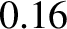(8)

It is apparent that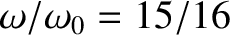is the motion's angular frequency: that is, the frequency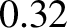converted into radians per second. (The units ofare hertz--otherwise known as cycles per second--whereas the units ofare radians per second. One cycle per second is equivalent toradians per second.) Finally, the phase angle,, determines the times at which the oscillation attains its maximum displacement,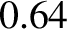. In fact, because the maxima ofoccur at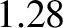, where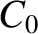is an arbitrary integer, the times of maximum displacement are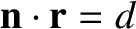(9)

Varying the phase angle shifts the pattern of oscillation backward and forward in time. (See Figure 3.)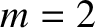Table 1: Simple harmonic oscillation.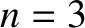0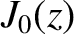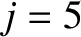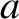0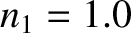0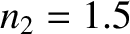0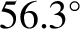0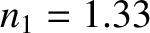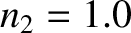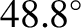0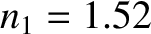0

Table 1 lists the displacement, velocity, and acceleration of the mass at various key points on the simple harmonic oscillation cycle. The information contained in this table is derived from Equation (3). All of the non-zero values shown in the table represent either the maximum or the minimum value taken by the quantity in question during the oscillation cycle.

As we have seen, when a mass on a spring is disturbed it executes simple harmonic oscillation about its equilibrium position. In physical terms, if the mass's initial displacement is positive (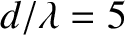) then the restoring force is negative, and pulls the mass toward the equilibrium point (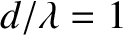). However, when the mass reaches this point it is moving, and its inertia thus carries it onward, so that it acquires a negative displacement (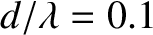). The restoring force then becomes positive, and pulls the mass toward the equilibrium point. However, inertia again carries it past this point, and the mass acquires a positive displacement. The motion subsequently repeats itself ad infinitum. The angular frequency of the oscillation is determined by the spring constant,, and the system inertia,, via Equation (6). On the other hand, the amplitude and phase angle of the oscillation are determined by the initial conditions. To be more exact, suppose that the instantaneous displacement and velocity of the mass atare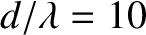and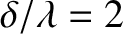, respectively. It follows from Equation (3) that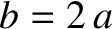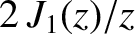(10)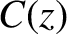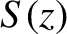(11)

Here, use has been made of the trigonometric identities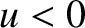and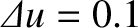. (See Appendix B.) Hence, we deduce that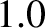(12)

and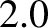(13)

because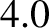and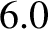. (See Appendix B.)

The kinetic energy of the system, which is the same as the kinetic energy of the mass, is written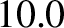(14)

The potential energy of the system, which is the same as the potential energy of the spring, takes the form (Fitzpatrick 2012)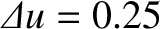(15)

Hence, the total energy is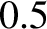(16)

because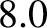and. According to the previous expression, the total energy is a constant of the motion, and is proportional to the amplitude squared of the oscillation. Hence, we deduce that the simple harmonic oscillation of a mass on a spring is characterized by a continual back and forth flow of energy between kinetic and potential components. The kinetic energy attains its maximum value, and the potential energy its minimum value, when the displacement is zero (i.e., when). Likewise, the potential energy attains its maximum value, and the kinetic energy its minimum value, when the displacement is maximal (i.e., when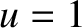). The minimum value of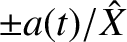is zero, because the system is instantaneously at rest when the displacement is maximal.Next: Simple Harmonic Oscillator Equation Up: Simple Harmonic Oscillation Previous: Simple Harmonic Oscillation
Richard Fitzpatrick 2013-04-08